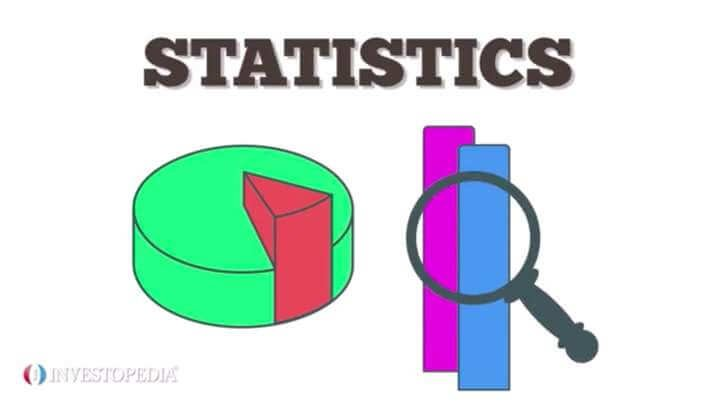# Statistics handwritten Notes & Question Bank for Jee mains 2020

Mathematics is the most vital parts of the entrance in fact, an indispensible part of human race. Chemistry, Physics and Mathematics have an equal weightage in the JEE but Mathematics has an edge over the other two as in case of a tie in the overall score of two or more candidates, the score in mathematics is considered to be the deciding factor. The subject is like the king of science which lays the foundation for Physics and Chemistry. Topics like calculus, differential equations and quadratic equations are used in almost all the questions of physics and chemistry.Jee mains syllabus is a perfect blend of easy and difficult topics. Some topics like probability, trigonometry, differential calculus, circles and straight lines in coordinate geometry, permutations and combinations in algebra are always easy to crack jee. Those days are gone when students used to spend hours in attempting one question. This is an era of multiple choice questions. Mathematics questions in the JEE test a student’s acquired knowledge as well as his aptitude.

STATISTICS SYLLABUS for Jee Mains

Measures of Dispersion: Calculation of mean, median, mode of grouped and ungrouped data calculation of standard deviation, variance and mean deviation for grouped and ungrouped data.

Every year 1 question comes from this chapter in jee mains

MATHS EASY AND IMPORTANT CHAPTERS ( JEE MAINS 2020 )

1. Statistics- 4 marks
2. Height and Distance- 4 marks
3. Mathematical Reasonong- 4marks
4. Matrices and Determinant- 8 marks
5. Sets,Relation and function – 8 marks
6. Vector and 3D– 8 marks